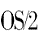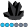OS2Rules
Obsidian | Level 7

## GBARLINE with Regression

Hi All:

Is it possible to use regression when creating the GBARLINE PLOT?

I have a symbol statement but it seems to ignore this.

1 ACCEPTED SOLUTION

Accepted Solutions

## Re: GBARLINE with Regression

The fundamental problem with doing regression lines in bar charts (and therefore bar-line charts)

is that the bars are considered to be evenly-spaced (character) variables, rather than treated

as a proportionally-spaced numeric axis.

To plot a regression line, you'd want to use a Gplot with a numeric axis.  You can then 'fake'

the bars in the Gplot by using the 'needle' interpolation.

Here is a GBarline example I've modified to use Gplot, and do a regression line:

title1 "NYSE Closing Price and Volume - 2002";
data nyse;
format Day date7.;
format High Low Close comma12.;
format Volume comma12.;
input Day date7. High Low Close Volume;
datalines;
01AUG07 10478.76 10346.24 10426.91 1908809
02AUG07 11042.92 10298.44 10274.65 1807543
05AUG07 10498.22 10400.31 10456.43 1500656
06AUG07 10694.47 10636.32 10762.98 1498403
07AUG07 10801.12 10695.13 10759.48 1695602
run;

symbol1 value=none interpol=needle width=15 color=blue;
symbol2 value=dot interpol=rl color=black;

axis1 offset=(15,15);

proc gplot data=nyse;
plot volume*day=1 / haxis=axis1;
plot2 close*day=2 / haxis=axis1;
run;

2 REPLIES 2

## Re: GBARLINE with Regression

The fundamental problem with doing regression lines in bar charts (and therefore bar-line charts)

is that the bars are considered to be evenly-spaced (character) variables, rather than treated

as a proportionally-spaced numeric axis.

To plot a regression line, you'd want to use a Gplot with a numeric axis.  You can then 'fake'

the bars in the Gplot by using the 'needle' interpolation.

Here is a GBarline example I've modified to use Gplot, and do a regression line:

title1 "NYSE Closing Price and Volume - 2002";
data nyse;
format Day date7.;
format High Low Close comma12.;
format Volume comma12.;
input Day date7. High Low Close Volume;
datalines;
01AUG07 10478.76 10346.24 10426.91 1908809
02AUG07 11042.92 10298.44 10274.65 1807543
05AUG07 10498.22 10400.31 10456.43 1500656
06AUG07 10694.47 10636.32 10762.98 1498403
07AUG07 10801.12 10695.13 10759.48 1695602
run;

symbol1 value=none interpol=needle width=15 color=blue;
symbol2 value=dot interpol=rl color=black;

axis1 offset=(15,15);

proc gplot data=nyse;
plot volume*day=1 / haxis=axis1;
plot2 close*day=2 / haxis=axis1;
run;OS2Rules
Obsidian | Level 7

## Re: GBARLINE with Regression

Robert:

That is exactly what I wound up doing.  I was just hoping that htere was some way for the GBARLINE to honour the SYMBOL statement and use the I=RL.

I found out (after a quick call to tech support) that the GBARLINE will only use I=JOIN, no matter what you code.

I would rather use a VBAR chart and overlay the regression line with ANNOTATE, but we don't have SAS STAT here and my statistics are rather rusty.

Thanks again.

Discussion stats
• 2 replies
• 686 views
• 0 likes
• 2 in conversation